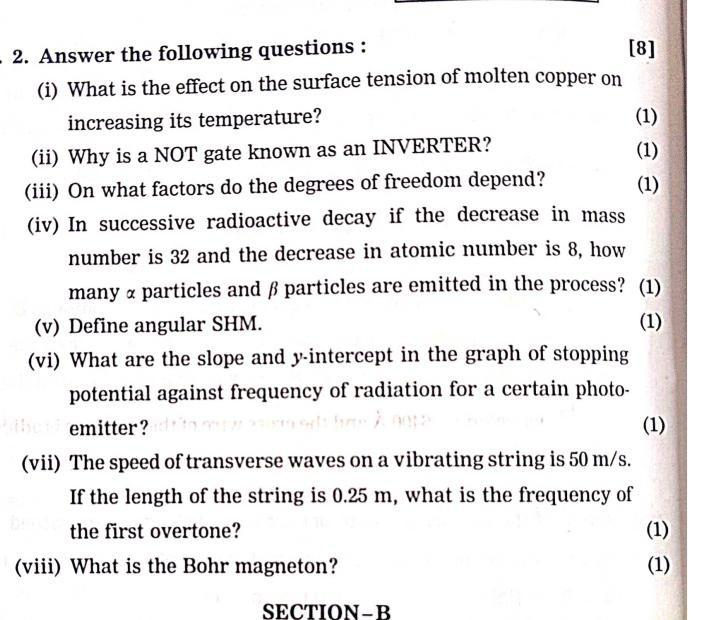Question:

#  - 2. Answer the following questions : (i) What is the effect on the surface tension of molten copper on increasing its tempe- 2. Answer the following questions : (i) What is the effect on the surface tension of molten copper on increasing its temperature? (1) (ii) Why is a NOT gate known as an INVERTER? (1) (iii) On what factors do the degrees of freedom depend? (1) (iv) In successive radioactive decay if the decrease in mass number is 32 and the decrease in atomic number is 8, how many a particles and ß particles are emitted in the process? (1) (v) Define angular SHM. (1) (vi) What are the slope and y-intercept in the graph of stopping potential against frequency of radiation for a certain photo- emitter? (1) (vii) The speed of transverse waves on a vibrating string is 50 m/s. If the length of the string is 0.25 m, what is the frequency of the first overtone? (1) (viii) What is the Bohr magneton? (1) SECTION-B